This is an jupyter notebook. Lectures about Python, useful both for beginners and experts, can be found at http://scipy-lectures.github.io.

Open the notebook by (1) copying this file into a directory, (2) in that directory typing jupyter-notebook and (3) selecting the notebook.

# Blind Source Separation¶

## Independent Component Analysis¶

A notebook by Shashwat Shukla and Dhruv Ilesh Shah

Required Packages: Python(2.7+), NumPy, SciPy Toolkit, Matplotlib

In this tutorial we will learn how to solve the Cocktail Party Problem using Independent Component Analysis(ICA). We will first take a look at Principle Component Analysis(PCA). The limitations of PCA will naturally lead to an understanding of what ICA does.

# Overview¶

## The Cocktail Party Problem(CPP)¶

So what is the Cocktail Party Problem? Imagine you are at a party where a lot of different conversations are happening in different parts of the room. As a listener in the room, you are receiving sound from all of these conversations at the same time. And yet, as humans, we possess the ability to identify different threads of conversation and to focus on any conversation of our choice. How do we do that? And how can we program a computer to do that? So this is essentially the Cocktail Party Problem: Given m sources(conversations at the party for example), and some number of sound receivers, separate out the different signals. (We will talk about how many receivers we need later.)

We need to make some mathematical assumptions and also phrase the problem more formally.

### The data¶

So first of all, our signals here are the sounds coming from different sources. At every (uniformly spaced) discrete interval of time we record m samples, one at each of our m microphones.

Note the implicit assumptions that we have made here:

1) There are as many microphoneses as there are independent conversations(sources) going on in the room. This assumption allows us to come up with a method to retrieve all the m independent signals. We can say that our system is critically determined(and is not under- or over- determined). Henceforth, we shall only consider this case in the tutorial.

2) Each microphone records a reasonably distinct combination of the independent signals. This simply amounts to not keeping two microphones too close to each other. Due to practical computational limits (see floating point math), it is always best to have easily distinguishable recordings.

How are we recording this data? We simply record the amplitude of the sound at each instant. Recording the pressure amplitude is a convenient thing to do(and is what a microphone does. A transducer then converts the pressure amplitude to a voltage). Note that we are recording the signals at discrete intervals of time (at a rate assumed to be greater than the Shannon Sampling rate)and will be working only in the time domain with these discrete signals.

One very important thing: We assume that the sound that any receiver records is a linear combination of sounds from the different sources. This is a reasonable assumption to make as pressure adds linearly. Each receiver will receive a different linear combination: If the first receiver is closer to a particular speaker than the second receiver, then the linear weight of this speaker will be proportionately higher for the first receiver.Courtesy: http://blog.csdn.net/muzilanlan/article/details/45917627

We further assume that each source is statistically independent with respect to all the other sources. We will look at a mathematical interpretation of statistical independence of two signals later. Within the context of the Cocktail Party parable an intuitive understanding of this assumption follows naturally, as the conversations happening in different parts of the room are independent of each other. Hence, knowing the signal at a particular instant from one source does not allow us to predict the value of the signal from any other source at that instant. They are independent variables.

This is the key assumption in Blind Source separation that allows to solve the problem.

We are also making one vital assumption about the sources of the signals: that they are non-Gaussian. We will look at what that means and why it matters in the section on Statistical Independence.

### The math¶

We will index our microphones from 1 to m.

The signal received by the microphone labelled i over the entire time of recording will be denoted by $X_{i}$. A particular sample of this recorded signal, recorded at the time index j will then be denoted by $X_{i}^{j}$.

Hence, if the samples of the signals recorded over time be N, then $X_{i}$ can be seen to be a row vector in N-dimensional space. It's jth element is given by $X_{i}^{j}$.

We had said that we have m microphones. Hence i in the above description ranges from 1 to m.

If we stack up these row vectors, we will get an m x N matrix whose ith row corresponds to the samples recorded by a particular microphone. A 'vertical slice' of this matrix, i.e a column corresponds to all the samples recorded at a given instant of time, indexed by the indices of the corresponding microphone.

Let us call this data matrix X.

To reiterate, $X_{i}^{j}$ corresponds to the sound sample recorded by the ith mike at the time (indexed by) j.

Let us now similarly define matrices corresponding to the sources that we wish to finally recover. The indices for the independent sources also go from 1 to m.

Let $S_{i}$ denote the signal generated by the ith independent source that we wish to recover (the ith conversation in the room). It is defined as a row vector.

$S_{i}^{j}$ is then the jth time sample of this signal.

Again, we vertically stack up these row vectors to get a m x N matrix denoted by S.

Now that we have defined our data and the signals that we wish to retrieve, we will describe the (assumed) relationship between the two. Note that we had assumed that the independent sources add linearly to give the recorded signals.

This means that each $X_{i}$ is some linear combination of the vectors $S_{1}$ through $S_{m}$.

Putting it all together, we conclude that $X = AS$ ; for some m x m matrix A, called the mixing matrix.

Our objective is to then find an "un-mixing" matrix W that satisfies $S = WX$.

If we know this W, as we already have X, we can calculate S by a direct multiplication.Courtesy: http://www.nocions.org/research/independent-component-analysis-ica-/

### Outline of solution¶

Our objective is to find the matrix W. As we have assumed that the number of microphones is equal to the number of independent conversations, it turns out that the matrix A is invertible and hence W is just the inverse of A.

Hence, it suffices to find A. We will employ Singular Value Decomposition(SVD) on the matrix A.Courtesy: Wikipedia
Hence, $A = UDV^{*}$ for orthogonal matrices U, V* and diagonal matrix D.

Note that V* is the conjugate transpose of V. Here the conjugate transpose is equivalent to the transpose because we are only dealing with real signals and their linear combinations.

We will then determine each of U, D, V* by considering the covariance matrix of x and exploiting the independence of the source signals.

The details follow.

## Covariance¶

An important term in the concept of statistics is covariance, which is a measure of how much two random variables change together. Covariance provides a measure of the strength of the correlation between two or more sets of random variates. The covariance for two random variates X and Y, each with sample size N, is defined by the expectation value: $$cov (X,Y)=\langle(X-\mu_x)(Y-\mu_y)\rangle\\=\langle X \rangle \langle Y \rangle-\mu_x \mu_y$$ where $\mu_x = \langle X \rangle$ and $\mu_y = \langle Y \rangle$ are the respective means.
For uncorrelated variates, $\langle X Y \rangle = \langle X\rangle\langle Y\rangle$ and hence, $$cov(X,Y)=\langle XY\rangle-\mu_x \mu_y=\langle X\rangle\langle Y\rangle-\mu_x \mu_y=0$$ However, if the variables are correlated in some way, then their covariance will be nonzero. In fact, if $cov(X,Y)>0$, then Y tends to increase as X increases, and if $cov(X,Y)<0$, then Y tends to decrease as X increases. Note that while statistically independent variables are always uncorrelated, the converse is not necessarily true.

As you can see, covariance can be a good metric to analyse the dependence of two datasets. To read more about covariance, follow this link.

As a special case, substituting $X = Y$ gives $$cov(X,X) = \langle X^2 \rangle - \langle X \rangle ^2 \\ =\sigma^2_X$$ where $\sigma_X$ denotes the standard deviation. Thus, $cov(X,Y)$ reduces to the statistical variance for this case.

Given a dataset vector $X$ (nx1 vector), the covariance of $X$ is given by $$C_x= (X-\mu_x)(X-\mu_x)^T$$ This matrix $C_x$ has very interesting properties, which we will be exploiting in the upcoming sections. This document would be an interesting read.

Now that we have the necessary tools required, let us dive into some algorithms that involve manipulating the information matrix and separating them into components. The first such algorithm is the PCA.

## Principle Component Analysis¶

Principal Component Analysis (PCA) is a statistical procedure that uses an orthogonal transformation to convert a set of observations of possibly correlated variables into a set of values of linearly uncorrelated variables called principal components. The number of principal components is less than or equal to the number of original variables. This transformation is defined in such a way that the first principal component has the largest possible variance (that is, accounts for as much of the variability in the data as possible), and each succeeding component in turn has the highest variance possible under the constraint that it is orthogonal to the preceding components.

What does this mean? Let's visualise the problem at hand.
Let us say you are given a dataset of point in the two-dimensional plane like the plot given below.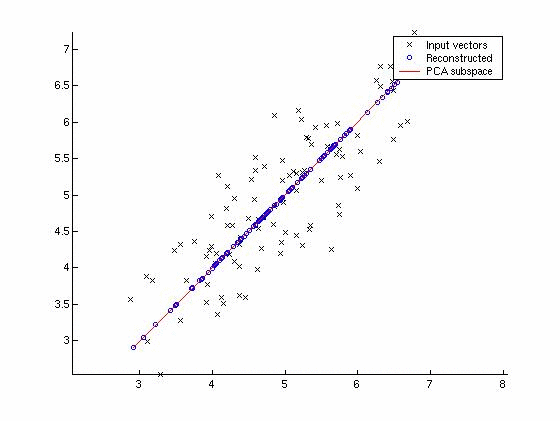Courtesy: Vision Dummy
The given dataset is two-dimensional and has a set of points arranged in a roughly elliptical form. What the PCA algorithm does is that it identifies the first principle component of the data, that is, the direction along which the variance of the data is maximum. This is the direction indicated by the red line. The second principle component is the one orthogonal to this, along the approximate minor axis of the dataset. Another such example of PCA on a 2D dataset is shown below.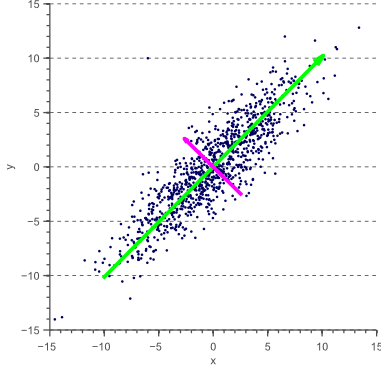Courtesy: [Center for Machine Perception, CTU, Prague](http://cmp.felk.cvut.cz/)
Here, the green line shows the vector corresponding to the first principle component, and pink shows the second component. To visualise how PCA can be applied on higher dimensional datasets, check out this page.

The PCA has various applications, some of which have been listed below.

• To identify the most important feature(s) of a multivariate function.
• Reduce dimensionality of data for saving memory, preserving maximum information.
• Speed up processes (regression, for example) by ignoring the features with low variance.
• As a pre-processing technique for further statistical methods, increasing efficiency.

Before we go ahead with understanding PCA, it is important to consider the following assumptions and limitation of PCA:

1. PCA assumes the dataset to be a linear combination of the variables.
2. There is no guarantee that the directions of maximum variance will contain good features for discrimination.
3. PCA assumes that components with larger variance correspond to interesting dynamics and lower ones correspond to noise.
4. Output vectors of PCA are orthogonal, which means that the principal components are orthogonal to each other.
5. PCA requires the data to be mean normalized and univariate, as it is highly susceptible to unscaled variables.
6. Since it assumes data to be uncorrelated and accurate, it is vulnerable to outliers and hence may produce incorrect results.

Here, we see that PCA breaks down the dataset into uncorrelated (hence, orthogonal) components.

### The Algorithm ¶

Principle component analysis relies on the property that the eigenvectors of the covariance matrix for a dataset $X$ represent a new set of orthogonal components that are the principle components of the dataset. Mathematically, given the covariance matrix $C_x$, the matrix $U = eig(C_x)$ which returns the eigenvectors is the desired matrix.
Another method to do this is singular value decomposition of the covariance matrix. In linear algebra, the singular value decomposition (SVD) is a factorization of a real or complex matrix. It is the generalization of the eigendecomposition of a positive semidefinite normal matrix (for example, a symmetric matrix with positive eigenvalues) to any $m \times n$ matrix via an extension of polar decomposition. The theory behind SVD is exhaustive and beyond the scope of this tutorial; you can find some interesting stuff here. For our use, SVD is called in a programming language like Matlab or Python as $$[U, S, V] = svd(C_x)$$ Such that $C_x$ satisfies $C_x = USV^T$. Note that $U$ here refers to the same matrix of orthogonal eigenvectors.

Thus, $U$ here is an $n\times n$ matrix with its column vectors as the eigenvectors of $C_x$.

• If we aim to perform data compression, define a matrix $U_{reduced} = U[:,1:k]$ which stores the first $k$ principle components. Thus, the required dataset with reduced dimensionality $k$ can be given by $X_{new} = U_{reduced}^TX$.
• If we do not want to compress data but simply express it in terms of principle/orthogonal components, we have $X_{new} = U^TX$

## PCA vs ICA¶

Now that we have seen what PCA is, let us see how it can be applied in our case - if at all.
We have seen that the basis on which PCA separates out the components is orthogonality and variance. Drawing parallels to the Cocktail Party Problem, there seems to be an issue. Orthogonality sure is a measure of independence of two datasets (vectors), but it is not the only measure and as it turns out, it is not applicable in the case of CPP. Two different sound signals need not be orthogonal, even if they are independent and this can be seen by simply generating a counter example. (Here, orthogonal refers to zero inner product.) The very fact that independent signals needn't be orthogonal eliminate the possibility of using PCA. Further, PCA identifies the separated components on the basis of variance, which according to Information Theory is a very shallow metric for decorrelating components. Statistical independence of the third or fourth order is generally preferred as a metric - what this means is that we must study deeper features and analyse correlations, but let's leave that for now.
Given below is the simplest way to illustrate how PCA fails due to the orthogonality condition on principle components.Despite the two branches being independent, PCA would not identify them so because it doesn't separate out independent components, but principle ones based on orthogonality and variance measures. ICA, as the name suggests, looks for independent components and not any dependent variable - hence avoiding side-effects! How it does this is by using a metric for statistical independence, described later, and then picking a model that minimizes this dependence. Before we can get to that, let's look at the pre-processing to be done on the data to ensure optimal results in ICA.

## Preprocessing for ICA¶

First, let us consider the basic statement of ICA. $$x = As \\ s = Wx$$ Where $s$ refers to the source signals, $A$, the mixing matrix and $x$, the signal we receive at microphones(say) and $W = A^{-1}$ Given below are the pre-processing stages performed:

• Centering: The most basic and necessary preprocessing is to center ${\bf x}$, i.e. subtract its mean vector ${\bf m = E\{x\}}$ so as to make ${\bf x}$ a zero-mean variable. This also implies that ${\bf s}$ is a zero-mean variable, as can be seen by taking expectation on both sides, above. This preprocessing is made solely to simplify the ICA algorithms: It does not mean that the mean could not be estimated. After estimating the mixing matrix ${\bf A}$ with centered data, we can complete the estimation by adding the mean vector of ${\bf s}$ back to the centered estimates of ${\bf s}$. The mean vector of ${\bf s}$ is given by ${\bf A}^{-1} {\bf m}$, where ${\bf m}$ is the mean that was subtracted in the preprocessing.
• Whitening: Another useful preprocessing strategy in ICA is to first whiten the observed variables. This means that before the application of the ICA algorithm (and after centering), we transform the observed vector ${\bf x}$linearly so that we obtain a new vector $\tilde{{\bf x}}$ which is white, i.e. its components are uncorrelated and their variances equal unity. In other words, the covariance matrix of $\tilde{{\bf x}}$ equals the identity matrix. A whitening transformation is a linear transformation that transforms a vector of random variables with a known covariance matrix into a set of new variables whose covariance is the identity matrix meaning that they are uncorrelated and all have variance unity. $$C_{\tilde{x}} = \tilde{x}\tilde{x}^T = I$$ The math behind whitening involves a greater understanding of eigenvectors and matrices, which we shall ignore for the purpose of this tutorial. Let us fast-forward to how whitening can be done on a given dataset. The whitening transformation is always possible. One popular method for whitening is to use the eigen-value decomposition (EVD) of the covariance matrix $C_{\tilde{x}} ={\bf E}{\bf D}{\bf E}^T$, where ${\bf E}$ is the orthogonal matrix of eigenvectors of $C_{\tilde{x}}$ and ${\bf D}$ is the diagonal matrix of its eigenvalues, ${\bf D}= \mbox{diag}(d_1,...,d_n)$. Note that $E\{{\bf x}{\bf x}^T\}$can be estimated in a standard way from the available sample $x(1),...,x(T)$. Whitening can now be done by $$\tilde{x} = ED^{-1/2}E^Tx$$ where the matrix ${\bf D}^{-1/2}$is computed by a simple component-wise operation as ${\bf D}^{-1/2}=\mbox{diag}(d_1^{-1/2},...,d_n^{-1/2})$. It is easy to check that now $C_{\tilde{x}}={\bf I}$.

It is important to note that the whitening transformation changes the matrix $A$ corresponding to the $x$, and hence $$\tilde{x} = ED^{-1/2}E^TAs = \tilde{A}s$$ The utility of whitening resides in the fact that the new mixing matrix $\tilde{{\bf A}}$ is orthogonal. How this affects the data is a little complicated to explain, but I will attempt to illlustrate it below.
Consider a two-dimensional dataset as given below, with uncorrelated/independent variables, along the sides of the parallelogram.[Swartz Center for Computational Neuroscience](http://sccn.ucsd.edu)
Evidently, this data has the two independent vectors as the sides of the parallelogram, but when passed into the algorithm, since the vectors are not orthogonal, they might end up showing unnatural dependencies which may not really exist. Hence, we perform whitening of the data to give something that looks like this:Courtesy: [Swartz Center for Computational Neuroscience](http://sccn.ucsd.edu)
Note that we haven't lost any information in the above transformation, nor have we created or destroyed any existing correlations. But this new dataset ($\tilde{x}$) is bound to perform better on ICA algorithms. In the rest of this tutorial, we assume that the data has been preprocessed by centering and whitening. For simplicity of notation, we denote the preprocessed data just by ${\bf x}$, and the transformed mixing matrix by ${\bf A}$, omitting the tildes.

## Statistical Independence¶

This section gives a brief description of statistical independence and it's interpretation in the context of this problem. Recall how we looked at an intuitive explanation. We said that voices are independent because listening to one won't allow us to predict the other.

To exploit this property mathematically, we construct the probability density functions(pdf) for each of the signals that our m microphones have recorded.

Why would we ever think of doing that? Well, for a number of pragmatic reasons. Statistical methods that work with density functions are very well developed and extremely powerful.

It also makes the math simpler. How you ask?

Well, notice that if we are representing a signal by it's probability density function alone, we are saying that at any given time, the value of the signal is simply a draw of a random variable with this particular pdf. (What this means is that we are basically discarding/not keeping track of the local correlation of the signal's contiguous values.)

In the context of the Cocktail Party Problem, we use pdfs to quantify statistical independence. There are many ways of quantifying the independence of two random variables if their pdfs are known. This is why we are working with probability density functions.

Here comes an extremely important point: While solving the Cocktail party problem, we assume that all the source signals that we wish to recover are non-Gaussian.

First of all, what do we mean by that? We mean that the pdf of each source signal is not a Gaussian function/distribution.

This might seem like an arbitrary and questionable assumption at first.

We invoke Information theory to justify our assumption. Simply put, there is a result in Information theory that says that the Gaussian distribution has the greatest possible entropy (for a fixed variance of the distribution). Entropy in our context is just disorder. The source signals do have order, as they contain lots of information. If they were purely Gaussian, then the signals would just sound like (Gaussian) noise.

We are not going to prove this result here. The mathematics is very involved and doesn't really shed any light on the present problem. However, an intuitive understanding of why this result should be right can be acquired by considering the famous Galton box(bean machine).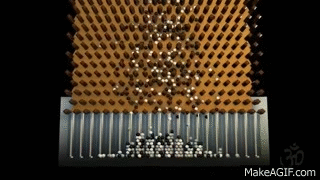Courtesy:http://tyulpinova.ru/normal_distribution/#
This is the epitome of a random process(that contains no information) and the fact that it has a Gaussian distribution is confirmation(if not justification) of the fact that the Guassian distribution has the greatest entropy.

Hence we assume that the original source signals have non-Gaussian distributions.

This revelation about the nature of the pdfs of the source signals actually allows us to solve the entire problem! We reiterate that each of the recorded signals is a linear sum of the original source signals. We now invoke the Central Limit Theorem.

The central limit theorem says that the pdf (of the average) of the sum of independent random variables, tends to a Gaussian distribution, as the number of random variables tend to infinity. Why is this important here? Well, we have assumed that the source signals are non-Gaussian independent variables and that the recorded signals are linear weighted sums of these non-Gaussian variables. By the Central Limit Theorem, the sum of non-Gaussian variables is more Gaussian than the individual variables. Hence, the recorded signals are more Gaussian than the source signals!

We finally have a way to recover our original signals. Remember that we are trying to linearly transform the recorded signals back to the source signals. Thus, now we have to just find the linear transformation that minimises the "Gaussian nature" of the transformed signals. The signals that have the least "Gaussian nature" simply correspond to our source signals.

Notice that I have put "Guassian nature" in quotes. This is because we have not yet quantified deviations from a Gaussian distribution. Indeed, this is precisely where FOBI and fastICA (and other ICA implementations) differ from one another. The steps that we have outlined in the previous sections apply to both. But the subsequent sections elucidate two different approaches to quantifying deviations from a Gaussian distribution and the details of the solutions to the Cocktail Party Problem formulated based on them.

## fastICA¶

The first method that we will be discussing is known as the fastICA which uses two important algorithms used widely in numerical analysis - gradient descent and fixed-point iteration. An understanding of both these is very important and hence we shall be discussing these before we encounter the actual algorithm. As a brief, we must estimate the matrix $W$ such that the source matrix $s = Wx$ has minimum 'statistical dependence'. To quantify the stastical dependence/independence, we use the concept of a cost function. We must choose the matrix $W$ which minimises the cost function, and hence maximises statistical independence. Fasten your seat belts, as we dive into the world of Machine Learning and tackle the topics one at a time, eventually solving the Cocktail Party Problem.

Let's assume we are given a function $f(x, y)$ , like the one given below, and an operation - say, to find the minima (or maxima) of the function over a domain.Courtesy: [http://www.network-graphics.com/images/calculus](http://www.network-graphics.com/images/calculus)

With a small amount of data, known function $f$ and just two variables $(x,y)$ itself, the problem can become difficult to solve algebraically. Simply computing minimas of a complex function comprising of exponential and trigonometric terms can become highly complex and computationally expensive; also, the solution is dependent on the type of function we wish to optimise, and here comes the need of a more generalised algorithm.

Come in, gradient descent! Let us now try to intuitively form an algorithm to solve such optimisation problems without any dependence of the function $f$, albeit some necessary conditions. Given that the function is continuously differentiable, we can claim that a minima is the point where the function is decreasin, no matter which direction you come from (similarly, maxima is where function is increasing no matter which direction you come from). In the given illustration , given a point $p_i$ or $(x,y)$ in the two-dimensional plane, and its gradient $\nabla f(p_i)$, if we could somehow follow the slope of the function, we could slide down all the way to a minima (or climb up to a maxima) and the value of function $f$ at that point would be the required optimised value.

A simple way to look at this is to imagine a hill, or a complex terrain just like the illustration. Say, you are sitting at a point on the terrain and wish to reach the bottommost/topmost point in your neighbourhood. For the minima of potential energy, for example, you could simply let gravity do the talking and slide down, in whichever direction it takes you, until you reach one of the minimas in your neighbourhood. What you just actuated, is known as gradient descent!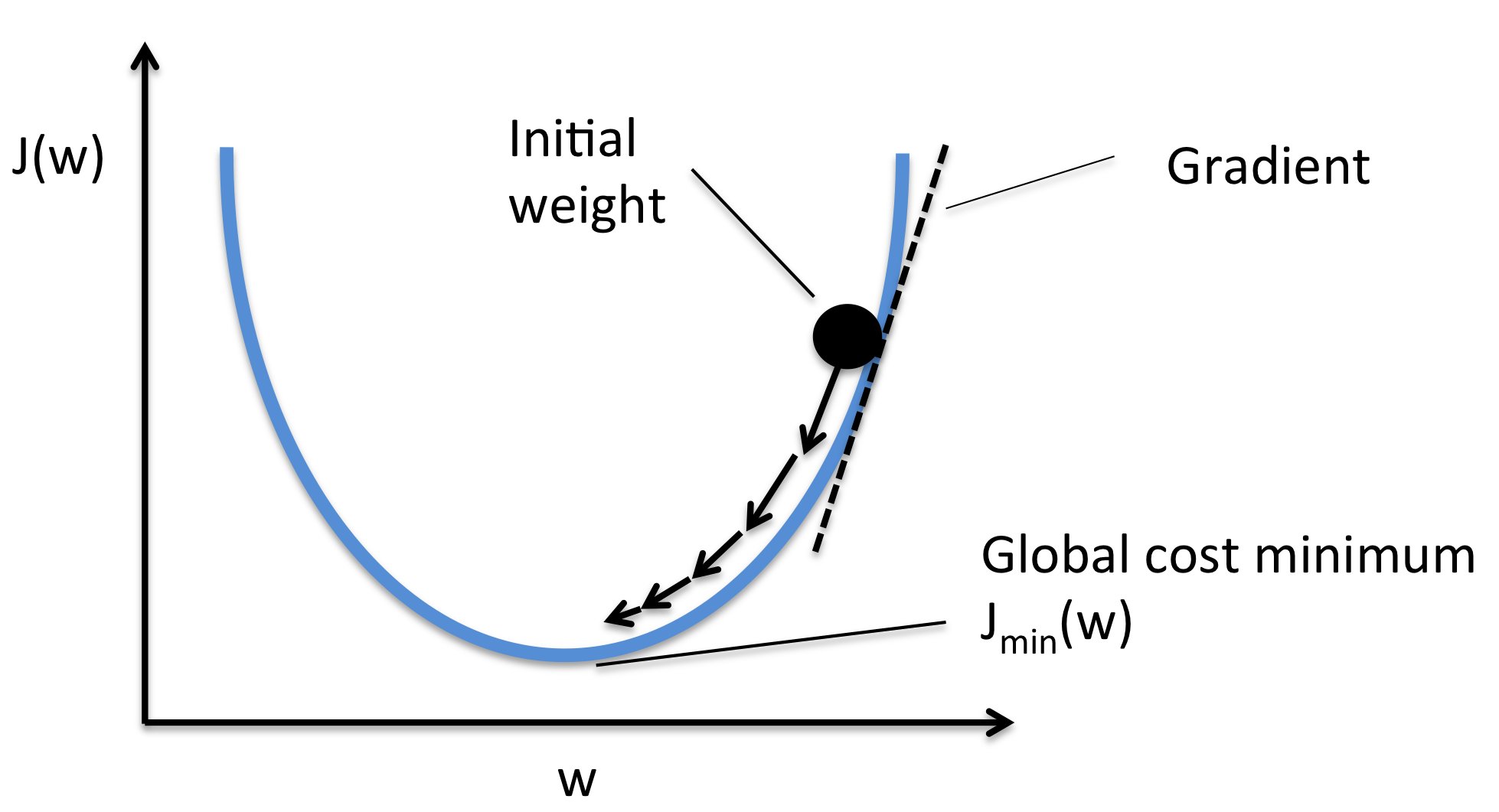Courtesy: [Sachin Joglekar's Blog](https://codesachin.files.wordpress.com)
In practice, it involves moving a small distance in the direction of gradient (inreasing or decreasing, based on the optimisation objective), until convergence. Note that we cannot be certain of landing at the actual minima, but we end up converging in the neighbourhood. The distance is controlled by a parameter $\alpha$, known as the step-size. Optimising this $\alpha$ is important because a large $\alpha$ can cause overshooting and failure in convergence, and a small $\alpha$ can slow down the process and make it computationally expensive. This can be tackled in two ways. * Running the algorithm multiple times and choosing an $\alpha$ that gives good results (a value of 0.3 to 2 should do fine). In fact, a good implementation of learning would be to provide the program with a range of $\alpha$s, say [0.01:10] on a logarithmic scale and making the system _learn_ the best parameter. _(This would require some background in Supervised ML and hence we leave this for you to explore!)_ * Another smart way to do this efficiently would be to make $\alpha$ dependent on the error of convergence (values of $f$ between two iteration), ideally the difference. This way, $\alpha$ automatically decreases as we get closer to the optimisation point. This _adaptive_ model is generally a better way to implement gradient descent as it guarantees fast convergence in a simplistic way. An important feature of gradient descent is that we can extend the algorithm to any n-dimesional space. We use 2-D to visualise it because visualising higher dimesional spaces can be complicated, almost impossible. But on extending the feature vector $X(x_1, ..., x_n)$ and a scalar function $f(X^i)$, this algorithm works just as well. **It must be noted that of the various drawbacks gradient descent may have, the most important one is perhaps the fact that it converges to _an_ optima, that is, a _local optima_ and not necessarily the global optima.** This can become a major issue with complicated functions, which may have multiple possible local optima. It is also possible that the algorithm converges to different optimas on different runs, depending on the starting point. ![An example of the issue.](http://www.holehouse.org/mlclass/01_02_Introduction_regression_analysis_and_gr_files/Image%20.png)
Courtesy: www.holehouse.org

In advanced machine learning, we use more complex optimisation tools in scientific packages like SciPy, MATLAB etc. (fminuc, fmincg etc.) We can ignore such complexities and assume that a run of gradient descent gives us the desired optima because of our choice of cost function (Coming Soon!).

That is all you need to know about gradient descent for this tutorial! Feel free to explore this topic further if you are interested. Gradient Descent has a wide range of applications in numerical analysis, the most exciting of which is in Machine Learning and Artificial Neural Networks!.

### Fixed-Point Iteration¶

Next up, we talk about another famous numerical solving technique known as the fixed-point iteration method. Given an expression $f(x)=0$, where $x$ can be a scalar or a vector, it is not always possible to solve it by standard methods. Consider the following equation. $$e^{x^2} - tan(x) + x = 0$$ It is not possible to solve such an equation directly, and thus we must use a method that can allow us to find a numeric solution. In general, the given function $f(x)$ can be anything and it would be nice if the method is independent of $f$. Consider the example of gradient descent above. We talked about what we would have to do, but how really would you take steps and move along the gradient? This is where the need of a solving method comes in. For now, let us consider solving an equation rather than an optimisation problem.

The FPI aims at solving the problem, as the name suggests, iteratively. The first step is to convert an equation of the form ${\bf f(x)=0}$ to the form ${\bf x = g(x)}$ so that we can update the parameter ${\bf x}$ directly. Let's tackle this with the help of an example. Let's say we want to find the roots of an equation $x^4 - x - 10 = 0$. We must first convert this into the form $x = g(x)$ to apply FPI. Some possible cases are $g_1(x)=x^4-10, g_2(x)=(x+10)^{1/4}, $$g_3(x)=(x+10)^{1/2}/x etc. It is intuitively evident that since we want convergence and run an iteration, the function g_i(x) better be a converging function, and henceg_1(x) is not a good function to use. The algorithm next involves iterating upto convergence, the following expression:$$ x_{n+1}=g(x_n) $$given the function g(x). We run this iteration untill the sequence converges, i.e., x_{n+1} - x_n < \epsilon, where \epsilon or tolerance is a small number. An important parameter that we must consider is the extent of convergence, or tolerance \epsilon. It defines how close two consecutive values of f must be, before we assume that convergence has occured. Choosing a value between 10^{-4} to 10^{-2} should work fine. Very small \epsilon may take too long to converge, or not converge at all, and a large \epsilon may give unsatisfactory results. Let us look at a simple example with the function defined above, and g(x) = (x+10)^{1/4}. We make an initial guess of x_0 = 1.0$$ x_1 = (x_0+10)^{1/4} = 1.82116 \\ x_2 = (x_2+10)^{1/4} = 1.85424 \\ x_2 = (x_1+10)^{1/4} = 1.85558 \\ x_3 = (x_2+10)^{1/4} = {\bf 1.85558} $$Similarly, starting with x_0 = 4.0, we get:$$ x_1 = (x_0+10)^{1/4} = 1.93434 \\ x_2 = (x_2+10)^{1/4} = 1.85866 \\ x_2 = (x_1+10)^{1/4} = 1.8557 \\ x_3 = (x_2+10)^{1/4} = 1.85559 \\ x_4 = (x_3+10)^{1/4} = {\bf 1.85558} $$defined as the normalized form of the fourth central moment of a distribution2 This way, we can solve an equation, indirectly, without worrying about the nature of the roots and the function itself. This is method in some very famous numerical methods like the Newton-Raphson's Method. Note that this method again finds one of the roots and can find different roots depending on seeding point. ### The Cost Function¶ We've talked all about optimising the function and solving for its optimal value and roots etc. But hey! In the problem that we were discussing where is this function? In this section we'll see how we can choose a function, whose optimisation gives us the desired result - separated sources. In any optimisation problem of this sort, we talk about a term called the cost, which (in the case of a minimisation) is analogous to the price you are paying by deviating from ideal results. A similar analogy can be thought of for maximisation. Hence, we must quantify the property statistical dependence and minimise it for the sources to be best separated. Note that the ideal case of perfect separation can generally not be attained due to various limitations. So, how do we quantify this dependence, or Gaussianity, as discussed in the last topic? • Kurtosis is defined as the normalized form of the fourth central moment of a distribution.$$ kurt(x)=E(x^4) - 3(E(x^2))^2 $$If we assume x to have zero mean \mu_x=E\{x\}=0 and unit variance \sigma^2_x=E\{x^2\}-\mu_x^2=1, then E\{x^2\}=1 and kurt(x)=E\{x^4\}-3. Kurtosis measures the degree of peakedness (spikiness) of a distribution and it is zero only for Gaussian distribution. Any other distribution's kurtosis is either positive if it is supergaussian (spikier than Gaussian) or negative if it is subgaussian (flatter than Gaussian). Therefore the absolute value of the kurtosis or kurtosis squared can be used to measure the non-Gaussianity of a distribution. However, kurtosis is very sensitive to outliers, and it is not a robust measurement of non-Gaussianity.Courtesy: [Harvey Mudd College](https://www.hmc.edu/) Lectures Hence, maximising this kurtosis function can be our optimisation objective! • Differential Entropy (Negentropy): Entropy is a very complex concept, which we wish to skip here, but as a fact, an important result in Information Theory states that the Gaussian Distribution the maximum entropy among all distributions over the entire real axis (-\infty,\infty). Thus, it can be used as a measure of Gaussianity. For more information on this, you can refer to this site. ### fastICA Algorithm¶ Pheww! That was long! Now let's finally take a look at the fastICA algorithm in a brief manner. 1. Obtain the data matrix x. 2. Subtract off the mean to center x. 3. Whiten the matrix x to obtain the matrix x^T. 4. Choose a random guess for the initial value of the de-mixing matrix {\bf w}. 5. Iterate the following:$$ w \gets E(xg(w^Tx)) - E(g'(w^Tx))w $$You will realise that this step is in fact the implementation of gradient descent using fixed-point iteration, as discussed above! The function g is a function used to capture higher order features of the matrix w^Tx and is similar to capturing the negentropy. 6. Normalize {\bf w}$$ w \gets w/{\|w\|}$$7. If not converged, go back to 2. Convergence can be judged by the fact that an ideal$w$would be orthogonal, and hence$w_i^Tw_{i+1}$would$\approx 1$. We thus use this condition to examine convergence. The gradient descent step uses a function$g$which can be a function like$cosh(x)$or$tanh(x)$which capture higher order correlations and hence we finally aim to maximise the function$\Sigma E(g(y_i))$where$y_i = {\bf w_i^Tx}$is a component of${\bf y = Wx}\$.

In :
%matplotlib inline
"""
Cocktail Party Problem solved via Independent Component Analysis.
The fastICA algorithm is implemented here,
using negentropy as a measure of non-gaussianity.
"""
# Import packages.
import matplotlib.pyplot as plt
from scipy import signal
import numpy as np
from scipy.io import wavfile
from scipy import linalg as LA
from numpy.random import randn as RNDN

def g(x):
out = np.tanh(x)
return out

def dg(x):
out = 1 - g(x) * g(x)
return out

# Dimension
dim = 2

# Input the data from the first receiver.
print "Sampling rate= ", samplingRate
print "Data type is ", signal1.dtype

# Convert the signal so that amplitude lies between 0 and 1.
# uint8 takes values from 0 through 255; sound signals are oscillatory
signal1 = signal1 / 255.0 - 0.5

# Output information about the sound samples.
a = signal1.shape
n = a
print "Number of samples: ", n
n = n * 1.0

# Input data from the first receiver and standardise it's amplitude.
signal2 = signal2 / 255.0 - 0.5

# x is our initial data matrix.
x = [signal1, signal2]

# Plot the signals from both sources to show correlations in the data.
plt.figure()
plt.plot(x, x, '*b')
plt.ylabel('Signal 2')
plt.xlabel('Signal 1')
plt.title("Original data")

# Calculate the covariance matrix of the initial data.
cov = np.cov(x)
# Calculate eigenvalues and eigenvectors of the covariance matrix.
d, E = LA.eigh(cov)
# Generate a diagonal matrix with the eigenvalues as diagonal elements.
D = np.diag(d)

Di = LA.sqrtm(LA.inv(D))
# Perform whitening. xn is the whitened matrix.
xn = np.dot(Di, np.dot(np.transpose(E), x))

# Plot whitened data to show new structure of the data.
plt.figure()
plt.plot(xn, xn, '*b')
plt.ylabel('Signal 2')
plt.xlabel('Signal 1')
plt.title("Whitened data")

Sampling rate=  8000
Data type is  uint8
Number of samples:  50000

Out:
<matplotlib.text.Text at 0xdd87240>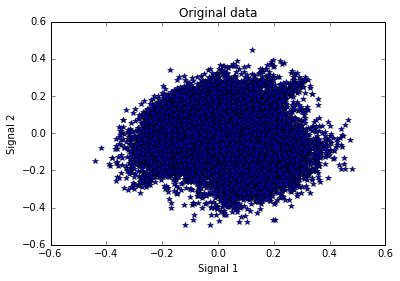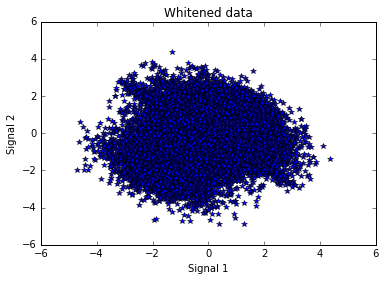In :
# Now that we have the appropriate signal,
# we proceed to implement fastICA on the source signal 'x'

# Creating random weight vector
w1 = RNDN(dim, 1)
w1 = w1 / LA.norm(w1)

w0 = RNDN(dim, 1)
w0 = w0 / LA.norm(w0)

# Running the fixed-point algorithm, with gradient descent
epsilon = 0.01  # Determines the extent of convergence
alpha = 1  # Step-size for gradient-descent

while (abs(abs(np.dot(np.transpose(w0), w1)) - 1) > epsilon):
w0 = w1
w1 = np.dot(xn, np.transpose(g(np.dot(np.transpose(w1), xn)))) / \
n - alpha * \
np.transpose(np.mean(np.dot(dg(np.transpose(w1)), xn), axis=1)) * w1
w1 = w1 / LA.norm(w1)

w2 = RNDN(dim, 1)
w2 = w2 / LA.norm(w2)

w0 = RNDN(dim, 1)
w0 = w0 / LA.norm(w0)

while (abs(abs(np.dot(np.transpose(w0), w2)) - 1) > 0.01):
w0 = w2
w2 = np.dot(xn, np.transpose(g(np.dot(np.transpose(w2), xn)))) / \
n - alpha * \
np.transpose(np.mean(np.dot(dg(np.transpose(w2)), xn), axis=1)) * w2
w2 = w2 - np.dot(np.transpose(w2), w1) * w1
w2 = w2 / LA.norm(w2)

# Forming the source signal matrix
w = np.transpose([np.transpose(w1), np.transpose(w2)])
s = np.dot(w, x)

# Plot the separated sources.
time = np.arange(0, n, 1)
time = time / samplingRate
time = time * 1000  # convert to milliseconds

plt.figure()
plt.subplot(2, 2, 1).set_axis_off()
plt.plot(time, s, color='k')
plt.ylabel('Amplitude')
plt.xlabel('Time (ms)')
plt.title("Generated signal 1")

plt.subplot(2, 2, 2).set_axis_off()
plt.plot(time, s, color='k')
plt.ylabel('Amplitude')
plt.xlabel('Time (ms)')
plt.title("Generated signal 2")

# Plot the actual sources for comparison.
orig1 = orig1 / 255.0 - 0.5  # uint8 takes values from 0 to 255

plt.subplot(2,2, 3).set_axis_off()
plt.plot(time, orig1, color='k')
plt.ylabel('Amplitude')
plt.xlabel('Time (ms)')
plt.title("Original signal 1")

orig2 = orig2 / 255.0 - 0.5  # uint8 takes values from 0 to 255

plt.subplot(2, 2, 4).set_axis_off()
plt.plot(time, orig2, color='k')
plt.ylabel('Amplitude')
plt.xlabel('Time (ms)')
plt.title("Original signal 2")

Out:
<matplotlib.text.Text at 0x9d3bf60>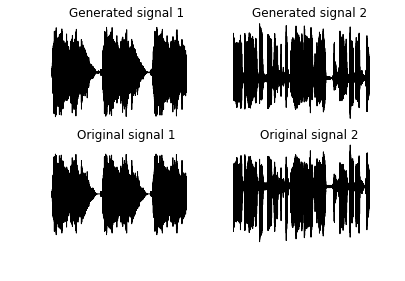But a good way to represent the audio files is through a spectrogram (short time fourier transform), google this if you dont know what this means. So we plot the spectrograms of our separation.

In :
plt.figure()
f, t, S = signal.spectrogram(s)
plt.pcolormesh(t, f, S)
plt.ylabel('Frequency [Hz]')
plt.xlabel('Time [sec]')
plt.title('Spectrogram of Output 1')

plt.figure()
f, t, S = signal.spectrogram(s)
plt.pcolormesh(t, f, S)
plt.ylabel('Frequency [Hz]')
plt.xlabel('Time [sec]')
plt.title('Spectrogram of Output 2')

# Storing numpy array as audio
wavfile.write('fastICA/out1.wav', samplingRate, np.transpose(s))
wavfile.write('fastICA/out2.wav', samplingRate, np.transpose(s))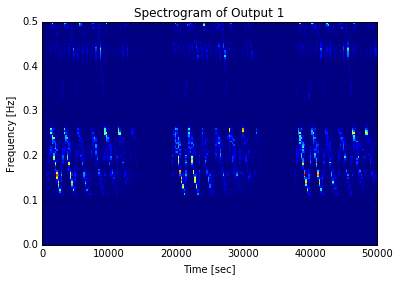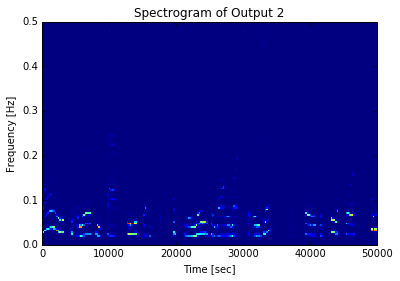In :
from IPython.display import Audio
print('Mixed Signal 1')
Audio("fastICA/mic1.wav")

Mixed Signal 1

Out:
In :
print('Mixed Signal 2')
Audio("fastICA/mic2.wav")

Mixed Signal 2

Out:
In :
print('Original separated signal 1')
Audio("fastICA/source1.wav")

Original separated signal 1

Out:
In :
print('Original separated signal 2')
Audio("fastICA/source1.wav")

Original separated signal 2

Out:
In :
print('Separated signal 1 (output)')
Audio("fastICA/out1.wav")

Separated signal 1 (output)

Out: